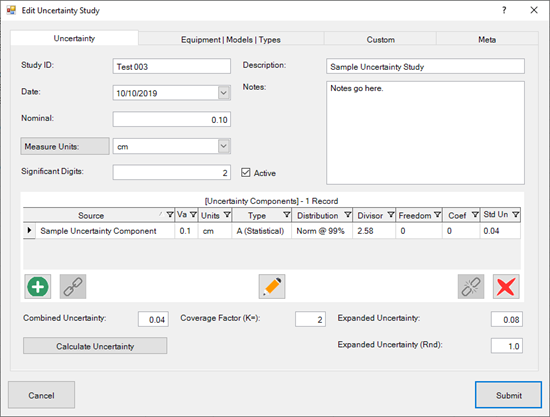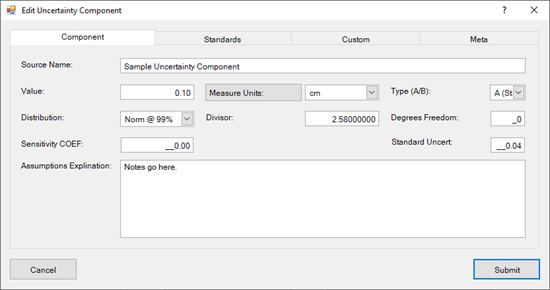# Measurement Uncertainty Studies

## Perform Uncertainty Analysis of a Measurement

The [Measurement] Uncertainty Studies grid (aka Uncertainty Budgets) in Calibration Control (our Calibration Management Software) is a simplified budget where Ape Software assumes no responsibility or liability for any unintentional errors.  It is the User’s responsibility to ensure calculations and data entry are accurate and to confirm the suitability for any particular purpose.

This budget is useful in situations where (a) each Component of Uncertainty is independent of the others, (b) the major Components are estimated with a high degree of accuracy, and (c) measurement results are measured directly or calculated with a linear formula using only multiplication or division (e.g., $W=V×I$).

Note that each Measurement Uncertainty Budget is conducted for a single Nominal measurement for a unique piece of Equipment.

### Uncertainty Study Dialog

• Study ID:  By default, the is a unique human-readable ID that can be edited.
• Date:  Date the Uncertainty Budget calculations were performed.
• Nominal:  Target value for the overall Uncertainty Budget analysis.
• Measure Units:  Measurement unit (e.g., inches, degrees, etc.) of the Nominal value for the Uncertainty Budget analysis.
• Significant Digits:  Number places to the right of the decimal.
• Description:  Description or title of the Uncertainty Budget analysis.
• Notes:  Any notes relevant to the Uncertainty Budget analysis.
• Combined [Standard] Uncertainty (${u}_{c}$):  Summation in quadrature (i.e., root sum of the squares) of all the Sources of Uncertainty in the grid. ${u}_{c}=\sqrt{\sum _{k=1}^{n}{{u}_{k}}^{2}}$
• Coverage Factor (e.g., $k=2$):  Used in the calculation of the Expanded Uncertainty and has a default value of $k=2$.  The Coverage Factor is a confidence level of the interval accounts for the variation in the estimate of the residual standard deviation, and is based on the assumption that the random errors have a normal distribution.
• Expanded Uncertainty ($U$):  The Expanded Uncertainty ($U$) assures a high level of confidence by re-scaling the Combined Uncertainty (${u}_{c}$) expressed with a different confidence level, e.g., 95 percent.  The re-scaling is accomplished by multiplying by the coverage factor (i.e., $U=k×{u}_{c}$).  In many cases, the coverage factor may be 2, which is typically used to approximate a 95% level of confidence.
• Expanded Uncertainty Rounded (${U}_{\mathrm{ce}}$):  The expanded uncertainty (${U}_{c}$) rounded to two significant digits.### Uncertainty Detail Dialog

Use the Detail dialog to describe each source of uncertainty (i.e., Component of Uncertainty) to be included in the overall Measurement Uncertainty Budget.

• Source Name:  Descriptive name for the Source of the Component of Uncertainty, which will be combined with other Components to create a single Uncertainty Budget.
• Value ($a$):  Numeric value related to the Unit of Measure.
• Unit of Measure: Units measured in such as inches, Fahrenheit, mph, etc.
• Type A/B:  Method used to estimate uncertainty either Type A, if estimated statistically, or Type B, if inferred through non statistical methods such as historical measurements, manufacturer specifications, calibration certificates, published data, mathematical formulas, or general experience.
• [Probability] Distribution:  The Probability Distribution selected dictates the Divisor (next field).  For example, a Normal 1s distribution has a Divisor of 1 where a Normal 2s distribution has a divisor of 2.  The following values for each Probably Distribution are defaulted into the Divisor field, which can be overridden.
 No Divisor Normal 1s = 1 Normal 2s = 2 Normal 3s = 3 Normal @ 99% = 2.58 Rectangle = $\sqrt{3}$ = 1.7321 Triangle = $\sqrt{6}$ = 2.4495 U-Shaped = $\sqrt{2}$ = 1.4142 Rectangle = $\sqrt{\mathrm{12}}$ = 3.4641
• Divisor ($d$):  The numeric value related to the Probability Distribution chosen.  This value is automatically set when choosing the Probability Distribution (previous field) but can be edited independently after choosing the Distribution.
• Degrees of Freedom ($v$):  For Type A uncertainties, use the d.f. for the corresponding standard deviations.  For Type B sources refer to calibration certificates or published reports.  In some instances, where the standard deviation must be estimated from scientific judgment or partial data, an infinite d.f. is normally assumed.
• Sensitivity Coefficient ($c$):  Used to describe how the Component of Uncertainty contributes to the Combined Uncertainty when a simple functional relationship does not exist between the input quantities and the measurement result.  For example, the unit of measure for the study may be in length units where an Uncertainty Component may be expressed in temperature or angular units.  Use this field to change the weighting of the Component from the default of 1 (one).
• Standard Uncertainty ($u$):  Calculated by dividing the Value ($v$) by the Divisor ($d$) and then multiplying by the Sensitivity Coefficient ($c$): $u=\frac{a}{d}×c$
• Assumptions Explanation:  Use this field to Explain any Assumptions made for the current Uncertainty Component.